Lecture 13
More on Chi-square

There are several loose ends on chi-square that I would like to tie up.

Simple Chi-square Problems
My discussion thus far has primarily focused on the 2 X 2 contingency table which looks at the goodness-of-fit or the independence of two dichotomous variables. I skipped a simpler example of chi-square which I would like to return to now.

A very easy and simple example of chi-square is when the frequencies of response to one dichotomous variable are compared. For this, we have just one variable that is tested. Examples of this are very common in the newspapers when polls are published. For instance, we might conduct a statewide survey of a sample of 300 hospital workers and ask the question "Do you think that staffing is adequate at your hospital, yes or no?" The following might be a hypothetical result:

 No Yes 180 120

The chi-square test asks if frequency of no responses is statistically larger (or different) from the frequency of yes responses. To compute this, we first need to know the expected frequency. Because we expect equal to imply a .5 chance of answering no or yes, we should expect half, or 150, of the survey participants to say yes or no. Thus we have this table of observed and expected frequencies:

 No Yes Oi 180 120 Ei 150 150

We then apply our usual chi-square formula:The degrees of freedom for this test is just the number of columns - 1 (c - 1 = 1). The critical value to exceed is equal at a = .05 is 3.84. Thus, respondents were significantly more likely to say no than yes to the question about adequate staffing.

This test can be conducted another way, using proportions. Usually survey responses are reported as the percentage (or, less often, proportion) of respondents indicating yes or no. These same results could be described as 60% indicating no and 40% indicating yes. The test to see whether these proportions are different is also appropriate for this example: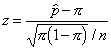where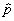is the obtained proportion and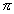(the Greek letter pi) is the proportion expected in the population (usually .5), and n is the sample size for the whole sample. Plugging in our results we get: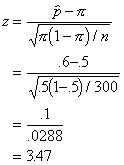Because chi-square and z are related, we should be able to show the equivalence of the two tests: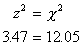And, within rounding error, this is the answer we got when we used the chi-square test of frequencies. The nice thing about the proportion test is that we can calculate a "margin of error" by computing our confidence intervals. I will just compute the UCL here.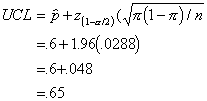This computation indicates that we can expect our survey to be accurate within about .048 or 5% on either side, because the confidence limits span from .55 to .65. This is the "margin of error" that is often reported with surveys in the media.

Of course, the same equivalence holds for the chi-square with at 2 X 2 contingency table and the test of two proportions that is presented in the book.

Post Hoc Follow-up Tests
Another chi-square topic concerns follow-up or post hoc tests when a larger contingency table (e.g., 3 X 3) is analyzed. Just like with the ANOVA test, the chi-square test is an "omnibus" test, because it is an overall test to see if there are differences between any of the cell frequencies. If just two of the cells in the design are significantly different, the omnibus test will be significant.

It is appropriate to conduct follow-up chi-square tests if one wants to discover which pairs of cells are significantly different. In conducting follow-up tests, some statisticians like to distinguish between "post hoc" follow-up tests and "a priori" tests. "Post hoc" means after the fact, and "a priori" means prior or planned ahead of time. The post hoc test is one that is not planned before the initial test and usually involves many or all possible comparisons. A priori tests on the other hand are typically fewer in number and test specific cell differences within the design that were predicted ahead of time.

For the post hoc tests, authors like to recommend a correction for problems with alpha inflation. In order to compensate for the alpha inflation problem, a researcher can set the alpha value required for significance to a lower value. The formula that is often used is called Bonferroni's correction and corrects for the number of chi-square tests and the d.f. involved in each test (actually this is a modified Bonferroni test suggested by Keppel). If we conducted 10 follow-up tests, each with d.f. = 1, we would use: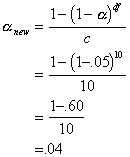So, we need to reset alpha to .04 to account for alpha inflation when conducting 10 single degree of freedom tests. Notice that alpha inflation is not that large for this many tests.

Within-subjects Chi-Square
There is a within-subjects version of the chi-square test. Imagine a study in which participants repeated the same measure twice, and the measure is a dichotomous one. In this case, the usual chi-square test is not appropriate, because we have a within-subjects design. Let's use the previous example of the hospital survey, but let's assume we first survey the 300 staff members before some important reorganization of staff is implemented. Then we resurvey them to see if their attitudes are changed. Notice that the frequency table looks similar to the 2 X 2 contingency table we had before. However, there is an important difference, and that is the fact that the measurements are repeated on the same group of 300. Our sample size is still 300 total.

 Time 2 No Yes Total Time No 80 100 180 1 Yes 10 110 120 Total 90 210 300

The test for this is simple. It is also a chi-square test, but it is called McNemar's chi-square test. If we label our cells like before, we can use a simple computational formula.

 Time 2 No Yes Time No a b 1 Yes c d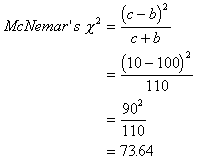This is a d.f.=1 test, and the critical value at a = .05 is 3.84. Thus, the change in opinions about staffing was wildly significant (sarcasm implied with the term "wildly").

Be careful about how you set up this table. Notice first that the cells represent a cross of people who said no on the first occasion and yes on the second occasion etc. Also, whether c is subtracted from b or a from d depends on how one sets up the table. One could list Yes first and No second, for instance. What you should keep in mind is that the test is based on the discordant cells (yes at Time 1 and no at Time 2; or no at Time 1 and yes at Time 2). Furthermore, it really does not matter what order the discordant cells are subtracted or added in the formula. We could have subtracted c from b instead. Because we square the difference, it makes no difference which is subtracted from which.

Multi-way Frequency Tables
Like factorial ANOVA, we can also examine two independent variables with chi-square. Our example in the 2 X 2 chi-square in the previous lecture involved gender differences in political affiliation. One can think of this as an independent variable of gender and a dichotomous dependent variable of political affiliation. We could complicate this by examining the same problem, but comparing regions (e.g., northern states to southern states). Our results might look like this:

Northern

 Females Males Total Democrats 50 70 120 Republicans 19 41 60 Total 69 111 180

Southern

 Females Males Total Democrats 70 30 100 Republicans 20 80 100 Total 90 110 200

One can think of the region variable as another independent variable. Does the effect of gender on political affiliation depend on the region the voter is from? In other words, is there an interaction between two independent variables. Notice, however, that the 2 X 2 X 2 contingency analysis is really analogous to the 2 X 2 ANOVA, because one of the 2's is the dichotomous outcome.

There are several ways to analyze this more complex design. One way is through a special chi-square that tests the equivalence of the two 2 X 2 contingency tables. Unfortunately, this test is not discussed or used very frequently, and is not available in SPSS. A second way to analyze this data is to compare the relative risk in the Northern region and the Southern region. That analysis compares two odds ratios and involves testing the interaction with logistic regression. We will briefly discuss this in a later section. A third way to analyze this design is to use something called "loglinear analysis." We will not be covering this topic in this class, but it is available in SPSS.